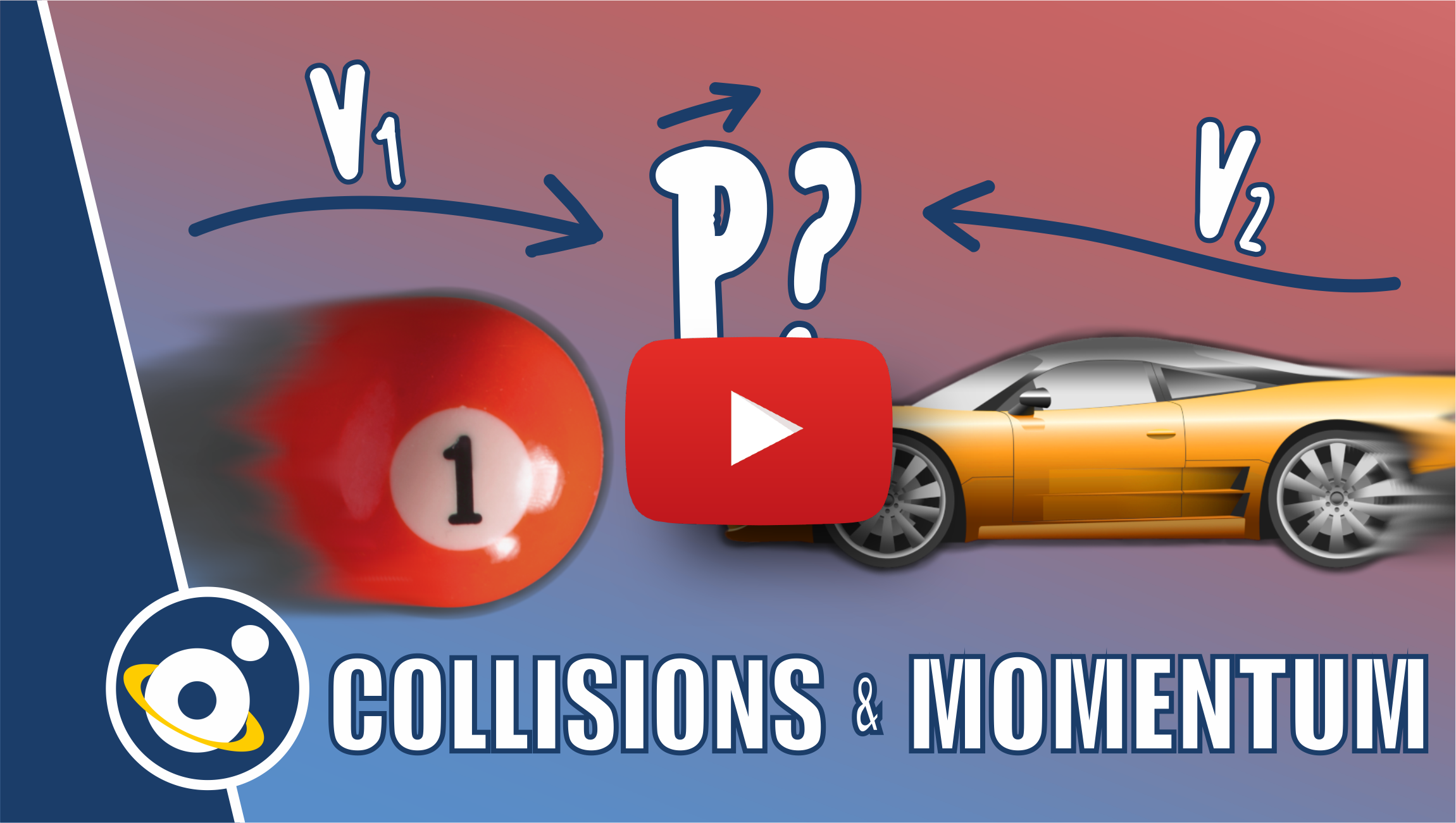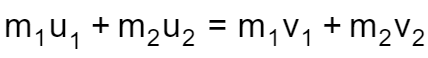# Conservation of Momentum Calculator

By Bogna Szyk and Steven Wooding
Last updated: Nov 25, 2021

The conservation of momentum calculator will help you in describing the motion of two colliding objects. Do you want to gain a better understanding of the law of conservation of momentum? Are you perplexed by the concepts of an elastic and inelastic collision? Or maybe you can't tell the difference between kinetic energy and momentum conservation principles? Whatever the reason, this article is here to help you.

Prefer watching rather than reading? Check out our video lesson on conservation of momentum here:## Law of conservation of momentum

The principle of momentum conservation says that for an isolated system, the sum of the momentums of all objects is constant (it doesn't change). An isolated system is a system of objects (it can be, and typically is, more than one body) that doesn't interact with anything outside the system. In such a system, no momentum disappears: whatever is lost by one object is gained by the other.

Imagine two toy cars on a table. Let's assume they form an isolated system - no external force acts on them, and the table is frictionless. One of the cars moves at a constant speed of 3 km/h and hits the second toy car (that remained stationary), causing it to move. You can observe that the first car visibly slows down after the collision. This result happened because some momentum was transferred from the first car to the second car.

## Elastic and inelastic collisions

We can distinguish three types of collisions:

• Perfectly elastic: In an elastic collision, both momentum and kinetic energy of the system are conserved. Bodies bounce off each other. An excellent example of such a collision is between hard objects, such as marbles or billiard balls.
• Partially elastic: In such a collision, momentum is conserved, and bodies move at different speeds, but kinetic energy is not conserved. It does not mean that it disappears, though; some of the energy is utilized to perform work (such as creating heat or deformation). A car crash is an example of a partially elastic collision - metal gets deformed, and some kinetic energy is lost.
• Perfectly inelastic: After an inelastic collision, bodies stick together and move at a common speed. Momentum is conserved, but some kinetic energy is lost. For example, when a fast-traveling bullet hits a wooden target, it can get stuck inside the target and keep moving with it.

You may notice that while the law of conservation of momentum is valid in all collisions, the sum of all objects' kinetic energy changes in some cases. The potential energy, however, stays the same (what is in line with the potential energy formula).

## How to use the conservation of momentum calculator

You can use our conservation of momentum calculator to consider all cases of collisions. To calculate the velocities of two colliding objects, simply follow these steps:

1. Enter the masses of the two objects. Let's assume that the first object has a mass of 8 kg, while the second one weighs 4 kg.
2. Decide how fast the objects are moving before the collision. For example, the first object may move at a speed of 10 m/s, while the second one remains stationary (speed = 0 m/s).
3. Determine the final velocity of one of the objects. For example, we know that after the collision, the first object will slow down to 4 m/s.
4. Calculate the momentum of the system before the collision. In this case, initial momentum is equal to 8 kg * 10 m/s + 4 kg * 0 m/s = 80 N·s.
5. According to the law of conservation of momentum, total momentum must be conserved. The final momentum of the first object is equal to 8 kg * 4 m/s = 32 N·s. To ensure no losses, the second object must have momentum equal to 80 N·s - 32 N·s = 48 N·s, so its speed is equal to 48 Ns / 4 kg = 12 m/s.
6. You can also open the advanced mode to see how the system's kinetic energy changed and determine whether the collision was elastic, partially elastic, or inelastic.

## FAQ

### What is the principle of conservation of momentum?

According to the principle of conservation of momentum, the total linear momentum of an isolated system, i.e., a system for which the net external force is zero, is constant.

### Under what circumstances is momentum conserved?

In order to conserve momentum, there should be no net external force acting on the system. If the net external force is not zero, momentum is not conserved.

### What is an example of conservation of momentum?

Recoiling of the gun when we fire a bullet from it is an example of conservation of momentum. Both the bullet and the gun are at rest before the bullet is fired. When the bullet is fired, it moves in the forward direction. The gun moves in the backward direction to conserve the total momentum of the system.

### What is the principle of working of a rocket?

The working principle of a rocket is based on the law of conservation of linear momentum. The fuel burnt in the rocket produces hot gas. The hot gas is ejected from the exhaust nozzle and goes in one direction. The rocket goes in the opposite direction to conserve momentum.

Bogna Szyk and Steven WoodingCollision type
Don't know
Object no. 1
Mass (m₁)
kg
Initial velocity (u₁)
m/s
Final velocity (v₁)
m/s
If you are trying to solve a problem that only has one object, maybe our impulse and momentum calculator would be more useful.
Object no. 2
Mass (m₂)
kg
Initial velocity (u₂)
m/s
Final velocity (v₂)
m/s
People also viewed…

### BMR - Harris-Benedict equation

Harris-Benedict calculator uses one of the three most popular BMR formulas. Knowing your BMR (basal metabolic weight), may help you make important decisions about your diet and lifestyle.

### Compton scattering

This Compton scattering calculator helps you to find the wavelength extension of the photon which is scattered on any particle.

### Thin Lens Equation

With this thin lens equation calculator you can find focal length of your lens.